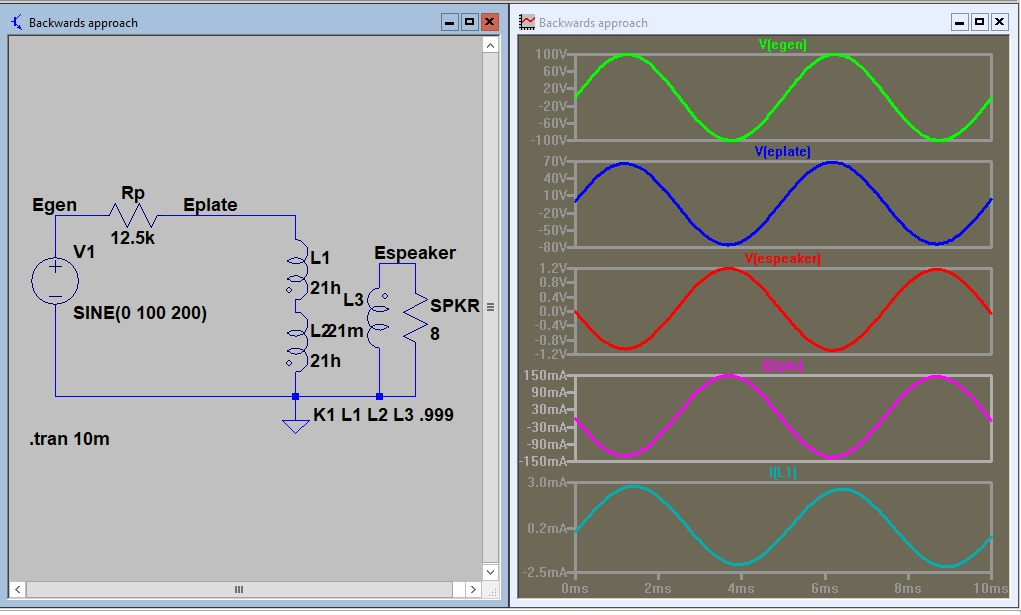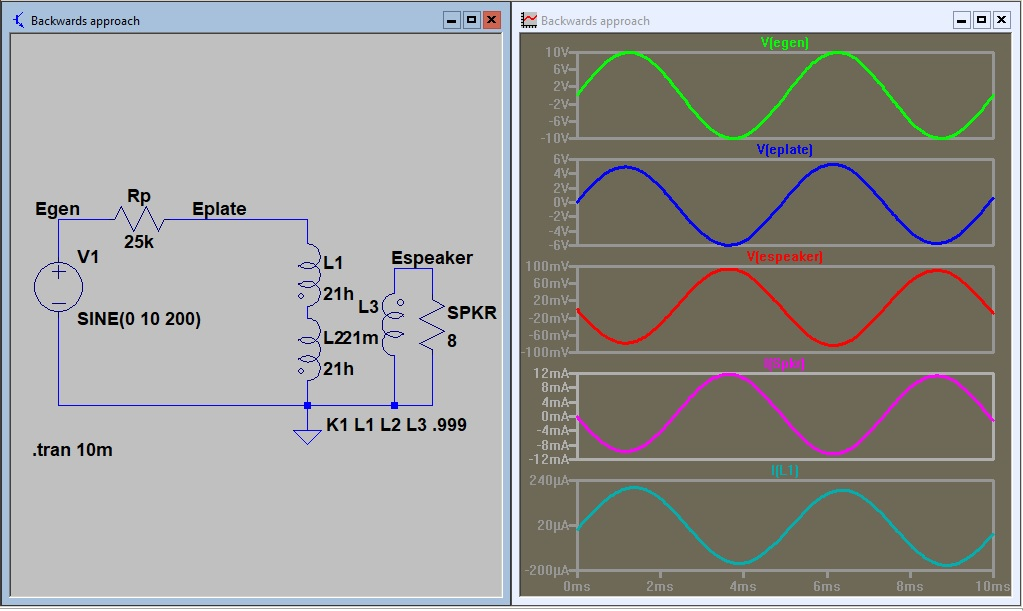## Friday, September 20, 2019

### Transformer - Z matching - what can we control?

I will make a series of screen shots with the transformer response displaying the circuit response to varying conditions and then pose the question what can we control and what do we have to work around? If we were having a custom made transformer made to our spec's we could just build a circuit and plug in the transformer but that is not the case. I measured a filament transformer inductance and will be using it as a typical example.This is the reference circuit. The first two readings show the circuit is a reasonable Z match.  It is dropping about 1/2 the signal in the plate circuit.decreasing the load decreased the internal signal drop.increasing the load increased the internal drop.Back to the original circuit. Rp is dropping half the signal so the circuit is matched. The only way to increase the output is to increase the signal (100 volts here).If I could lower the generator Z it would increase the output.Lowering the generator more gives more increase.Lowering the turns ratio increased the output.If you took the T725 challenge you can calculate the required signals for a 120 volt to 12 volt transformer to match your speaker.
The questions are:
1) What would the valve anode load resistance need to be to match a 10:1 turns ratio with a 16 ohm load?
2) What tube would match?
3) What signal swing would be required for 250mw output?